Feed Back Membership Ask Question Free Download Help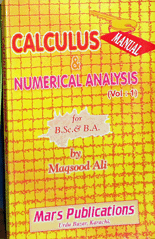TOPIC ARRANGEMENTS - COLLEGE MATHEMATICS

STEPS OF TOPICS' ARRANGEMENTS

In order to make the students understand, practice and grasp the topics thoroughly. They have been divided and arranged in the following :

i) DEFINITION :
In order to solve a problem of any topic it is vital to first understand the definition of the topic . That is why the definition of every topic is given in one or more ways in this book.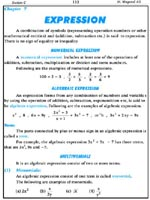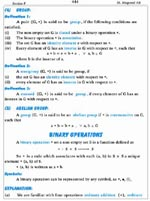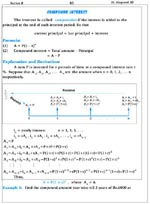ii) EXPLANATION:
It requires a lot of expertise , thorough study and deep knowledge to explain a topic of mathematics. The author of this book has used very easy language and understandable vocabulary , several diagrams, sketches , graphs and diagrams in different c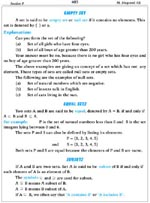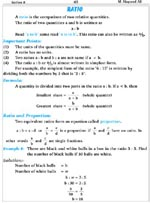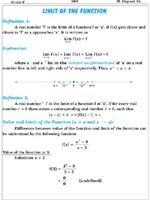olours so that the teachers and the students could grasp the topics easily.

iii) FORMULAS:
All the formulas related to a topic are given as well as the problems are solved by all those Formulas, which can be used to solve the problem.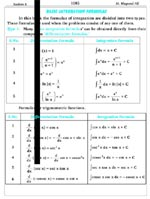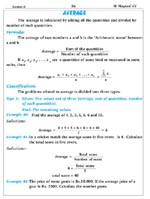iv) DIAGRAMS:
As diagrams are unavoidable to grasp the theme of the topics, therefore, 1600 diagrams have been included in this book of 1200 pages. Diagrams also play an important role to understand the objects of the real world mathematically. The author has used different colours in the diagrams as per need.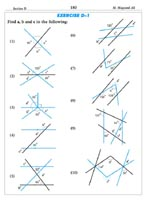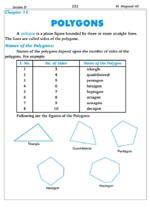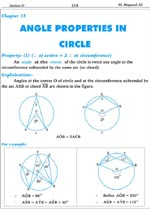v) SOLVED EXAMPLES:
Without solved examples , the Mathematics book may be called an incomplete book because the solved examples are the most important and the main part to understand Mathematics. By means of solved examples one can get an idea of importance and as well as the application of the topic. The author has arranged the solved examples so that any student could be able to understand the topic very easily. The author emphasized the following points in the solved examples:

• It can make easy to understand the topic.
• It can help to solve the problems related to the topic.
• It can help to understand the application as well as usage of the topic.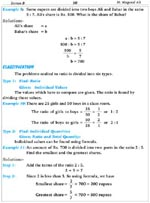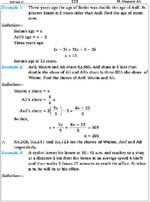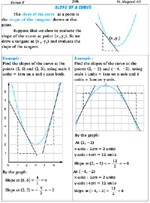vi) SHORTCUTS:
The most important factor for MCQs is the Time Factor. That is why the author has included all the shortcuts solutions of problems. These shortcuts help to solve the problems in the least amount of time. It is also helpful for the students of GRE , SAT or any type of Aptitude Test.

vii) MCQs:
At present, the MCQs are known as the best way of assessing the ability of students in the shortest time. Therefore, the author gave particular emphasis on MCQs so that the student could attain significant competency of mathematics in all respect. This book consists of about 1400 MCQs related to almost every topic of Mathematics.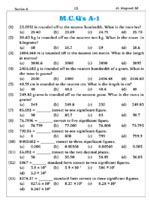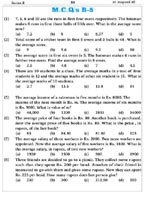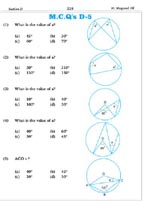viii) UNSOLVED PROBLEMS:
The author has arranged every exercise in easy to difficult order i.e. he started the Exercises with easy problems and went to difficult problems gradually. This way of practice of problems would enable student to solve every type of easy or difficult problems.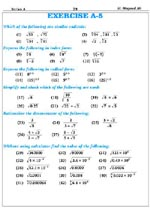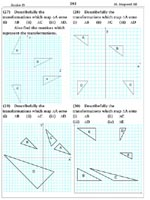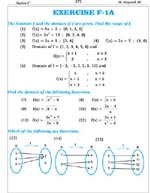@ Math Bunch. All rights reserved. Powered By UiTech